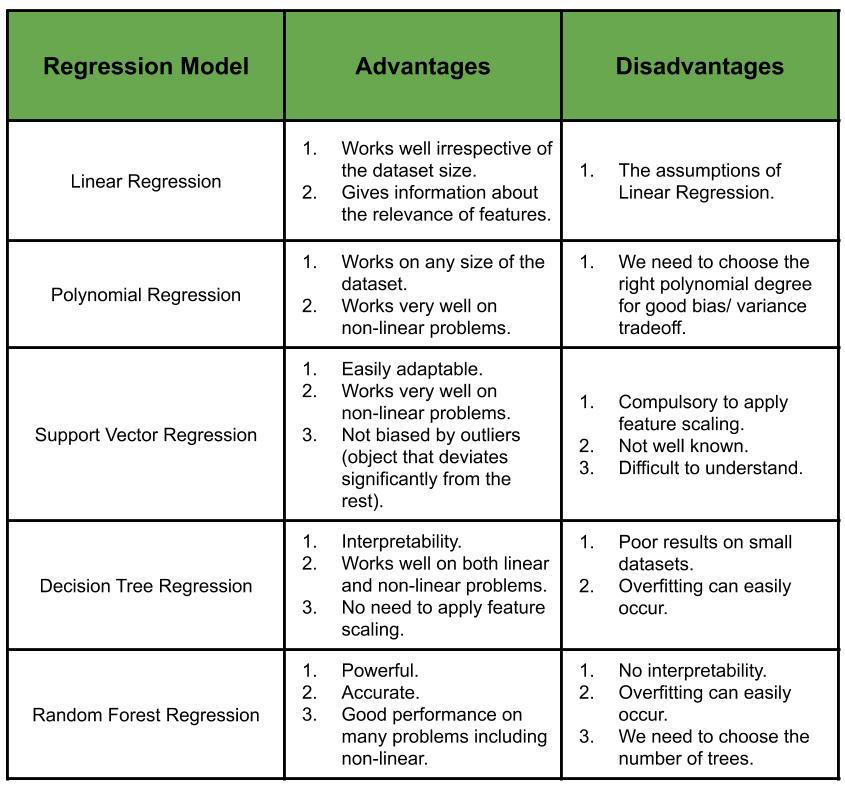• Last Updated : 17 Jul, 2020

Regression is a typical supervised learning task. It is used in those cases where the value to be predicted is continuous. For example, we use regression to predict a target numeric value, such as the car’s price, given a set of features or predictors ( mileage, brand, age ). We train the system with many examples of cars, including both predictors and the corresponding price of the car (labels).

Types of Regression Models:

Attention reader! Don’t stop learning now. Get hold of all the important Machine Learning Concepts with the Machine Learning Foundation Course at a student-friendly price and become industry ready.

• Simple Linear Regression is a linear regression model that estimates the relationship between one independent variable and one dependent variable using a straight line.
Example : Salary = a0 + a1*Experience ( `y = a0 + a1x `form ).
• Multiple Linear Regression is a linear regression model that estimates the relationship between several independent variables (features) and one dependent variable.
Example : Car Price = a0 + a1*Mileage + a2*Brand + a3*Age ( `y = a0 + a1x1 + a2x2 + ... + anxn` form )
• Polynomial regression is a special case of multiple linear regression. The relationship between the independent variable x and dependent variable y is modeled as an nth degree polynomial in x. Linear regression cannot be used to fit non-linear data (underfitting). Therefore, we increase the model’s complexity and use Polynomial regression, which fits such data better. ( `y = a0 + a1x1 + a2x12 + ... + anx1n` form )
• Support Vector Regression is a regression model in which we try to fit the error in a certain threshold (unlike minimizing the error rate we were doing in the previous cases). SVR can work for linear as well as non-linear problems depending on the kernel we choose. There is an implicit relationship between the variables, unlike the previous models, where the relationship was defined explicitly by an equation (coefficients are sufficient to balance the scale of variables). Therefore, feature scaling is required here.
• Decision Tree Regression builds a regression model in the form of a tree structure. As the dataset is broken down into smaller subsets, an associated decision tree is built incrementally. For a point in the test set, we predict the value using the decision tree constructed
• Random Forest Regression – In this, we take k data points out of the training set and build a decision tree. We repeat this for different sets of k points. We have to decide the number of decision trees to be built in the above manner. Let the number of trees constructed be n. We predict the value using all n trees and take their average to get the final predicted value for a point in the test set.How do we choose the right Regression Model for a given problem ?
Considering the factors such as – the type of relation between the dependent variable and the independent variables (linear or non-linear), the pros and cons of choosing a particular regression model for the problem and the Adjusted R2 intuition, we choose the regression model which is most apt to the problem to be solved.

My Personal Notes arrow_drop_up